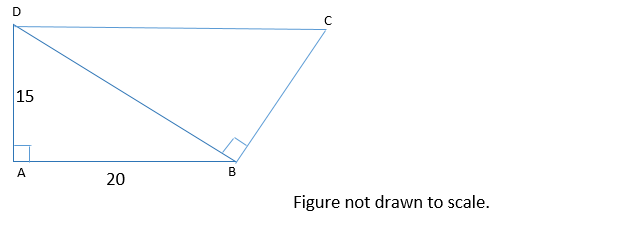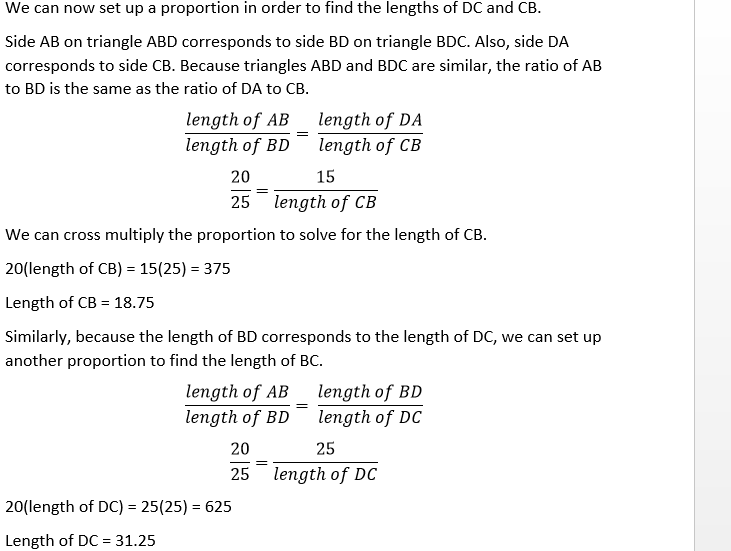# High School Math : How to find if right triangles are similar

## Example Questions

### Example Question #83 : Right Triangles

You have two right triangles that are similar.  The base of the first is 6 and the height is 9.  If the base of the second triangle is 20, what is the height of the second triangle?

23

35

33

25

30

30

Explanation:

Similar triangles are proportional.

Base1 / Height1 = Base2 / Height2

6 / 9 = 20 / Height2

Cross multiply  and solve for Height2

6 / 9 = 20 / Height2

6 * Height2=  20 * 9

Height2=  30

### Example Question #1 : Right TrianglesIn the figure above, line segments DC and AB are parallel. What is the perimeter of quadrilateral ABCD?

95

85

80

75

90

85

Explanation:

Because DC and AB are parallel, this means that angles CDB and ABD are equal. When two parallel lines are cut by a transversal line, alternate interior angles (such as CDB and ABD) are congruent.

Now, we can show that triangles ABD and BDC are similar. Both ABD and BDC are right triangles. This means that they have one angle that is the same—their right angle. Also, we just established that angles CDB and ABD are congruent. By the angle-angle similarity theorem, if two triangles have two angles that are congruent, they are similar. Thus triangles ABD and BDC are similar triangles.

We can use the similarity between triangles ABD and BDC to find the lengths of BC and CD. The length of BC is proportional to the length of AD, and the length of CD is proportional to the length of DB, because these sides correspond.

We don’t know the length of DB, but we can find it using the Pythagorean Theorem. Let a, b, and c represent the lengths of AD, AB, and BD respectively. According to the Pythagorean Theorem:

a2 + b2 = c2

152 + 202 = c2

625 = c2

c = 25

The length of BD is 25.We now have what we need to find the perimeter of the quadrilateral.

Perimeter = sum of the lengths of AB, BC, CD, and DA.

Perimeter = 20 + 18.75 + 31.25 + 15 = 85

### Example Question #1 : How To Find If Right Triangles Are Similar

A right triangle is defined by the points (1, 1), (1, 5), and (4, 1).  The triangle's sides are enlarged by a factor of 3 to form a new triangle.  What is the area of the new triangle?

None of the answers are correct

36 square units

81 square units

54 square units

108 square units